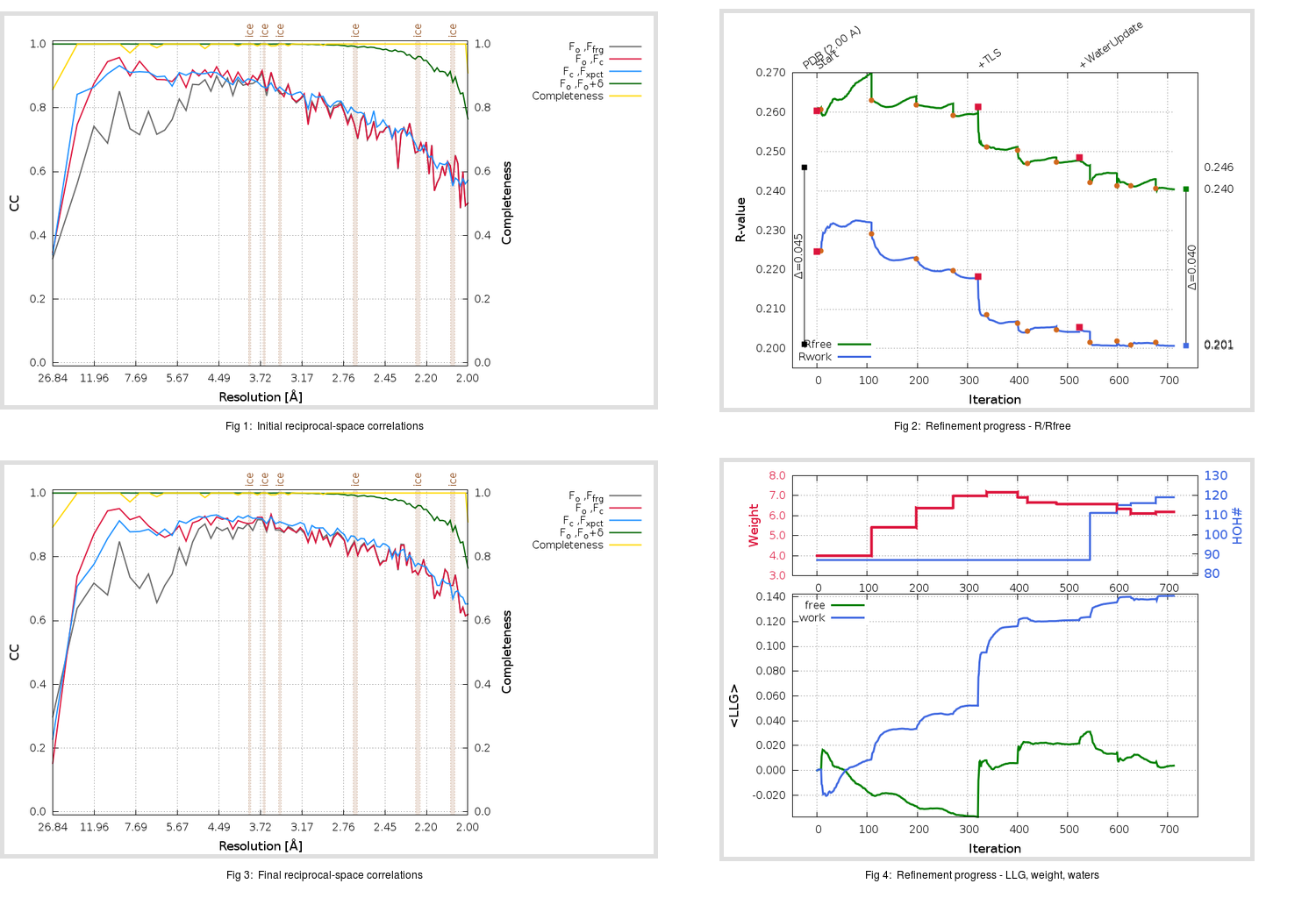Content:

```    Diffraction limits & principal axes of ellipsoid fitted to diffraction cut-off surface:
1.965         0.9226   0.0000   0.3859       0.997 a* + 0.080 c*
1.926         0.0000   1.0000   0.0000       b*
1.904        -0.3859   0.0000   0.9226      -0.705 a* + 0.709 c*
```

## Deposited

` `
 Date deposited Date data collection Resolution R, Rfree 20200219 20200211 2.00 0.1960 0.2460

Molprobity (CCP4 7.0 version) summary:

```Ramachandran outliers =   0.00 %
favored =  96.71 %
Rotamer outliers      =   0.00 %
C-beta deviations     =     0
Clashscore            =   4.06
RMS(bonds)            =   0.0040
RMS(angles)           =   1.08
MolProbity score      =   1.40
Resolution            =   2.00
R-work                =   0.1960
R-free                =   0.2460
```

```Number of waters      =    87

<B> (all atoms) =   52.38 ( sd =   14.84 ) for       2454 non-hydrogen atoms
<B>   (protein) =   52.28 ( sd =   14.93 ) for       2367 non-hydrogen atoms
<B>     (water) =   54.99 ( sd =   11.76 ) for         87 non-hydrogen atoms
<B>    (others) =    0.00 ( sd =    0.00 ) for          0 non-hydrogen atoms

B min/max       (all non-hydrogen atoms) =   26.05 /  111.54
B min/max   (protein non-hydrogen atoms) =   26.05 /  111.54
B min/max     (water non-hydrogen atoms) =   33.15 /   96.66
B min/max     (other non-hydrogen atoms) =    0.00 /    0.00
```

## BUSTER (re-)refinement

` `

Molprobity (CCP4 7.0 version) summary:

```Ramachandran outliers =   0.66 %
favored =  96.38 %
Rotamer outliers      =   1.90 %
C-beta deviations     =     0
Clashscore            =   2.78
RMS(bonds)            =   0.0121
RMS(angles)           =   1.56
MolProbity score      =   1.52
Resolution            =   1.99
R-work                =   0.2006
R-free                =   0.2404
```

```Number of waters      =   119

<B> (all atoms) =   49.25 ( sd =   11.01 ) for       2486 non-hydrogen atoms
<B>   (protein) =   49.02 ( sd =   11.00 ) for       2367 non-hydrogen atoms
<B>     (water) =   53.75 ( sd =   10.23 ) for        119 non-hydrogen atoms
<B>    (others) =    0.00 ( sd =    0.00 ) for          0 non-hydrogen atoms

B min/max       (all non-hydrogen atoms) =   30.82 /   97.27
B min/max   (protein non-hydrogen atoms) =   30.82 /   97.27
B min/max     (water non-hydrogen atoms) =   32.55 /   84.47
B min/max     (other non-hydrogen atoms) =    0.00 /    0.00
```

Refinement progression:Results:

` `
 File Remark 6M03_aB_refine.01_03_refine.pdb.gz exact refinement commands are in header 6M03_aB_refine.01_03_refine.mtz.gz including original deposited data and several re-refinement map coefficients 6M03_aB_refine.01_03_BUSTER_model.cif.gz including any non-standard compound restraints 6M03_aB_refine.01_03_BUSTER_refln.cif.gz